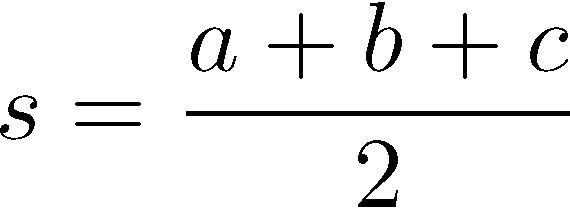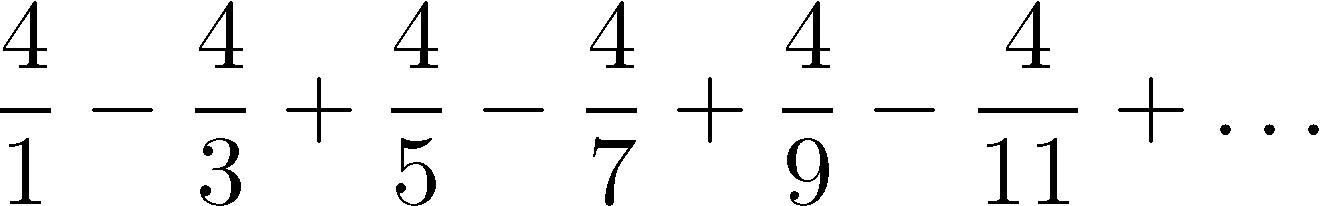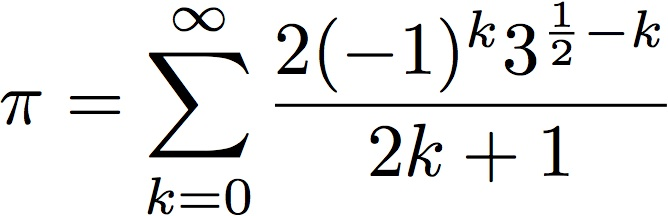# Homework 1 (Revised)

Due: October 10, 2019
Points: 100

1. (25 points) The Konditorei coffee shop sells coffee at \$10.50 a pound plus the cost of shipping. Each order ships for \$0.86 per pound + \$1.50 fixed cost for overhead. Write a program that calculates the cost of an order. Your program must prompt the user for the weight of the order in pounds.
To turn in: Please turn in the program in the file kondit.py.
2. (30 points) Write a program to calculate the area A of a triangle given the length of its three sides a, b, and c using this formulae-:wherePrompt the user for the lengths of the three sides, one per line.
To turn in: Please turn in the program in the file tri.py.
Revision: The previous version had input entered as three numbers on the same line, separated by commas. This revision has the user enter one number per line.

3. (45 points) Write a program that approximates the value of π by summing the terms of this series:The program is to prompt the user for n, the number of terms to sum, and then output the sum of the first n terms of this series. (If n is 1, output the value of the first term only.) Your program is also to print the absolute value of the difference between the approximation and the value of math.pi to see how accurate the approximation is.
To turn in: Please turn in the program in the file pi.py.

### Extra Credit

1. (20 points) Abraham Sharp developed an infinite sum that produces π:1. (15 points) Compute and print the resulting approximations to π for the first 5, 10, …, 50 terms. Each line of your output should look like this:
```After 5 terms, the approximation is 3.1428047456632134
```
Your number may differ; this is intended to show you the format of the output only.
2. (5 points) After what term does adding extra terms stop improving the approximation?

To turn in: Please turn in the program in the file sharp.py. If you answer the second part, put the number in a comment, clearly labeled, at the top of the file.Matt Bishop Office: 2209 Watershed Sciences Phone: +1 (530) 752-8060 Email: mabishop@ucdavis.edu
ECS 36A, Programming and Problem Solving
Version of October 7, 2019 at 1:24PM